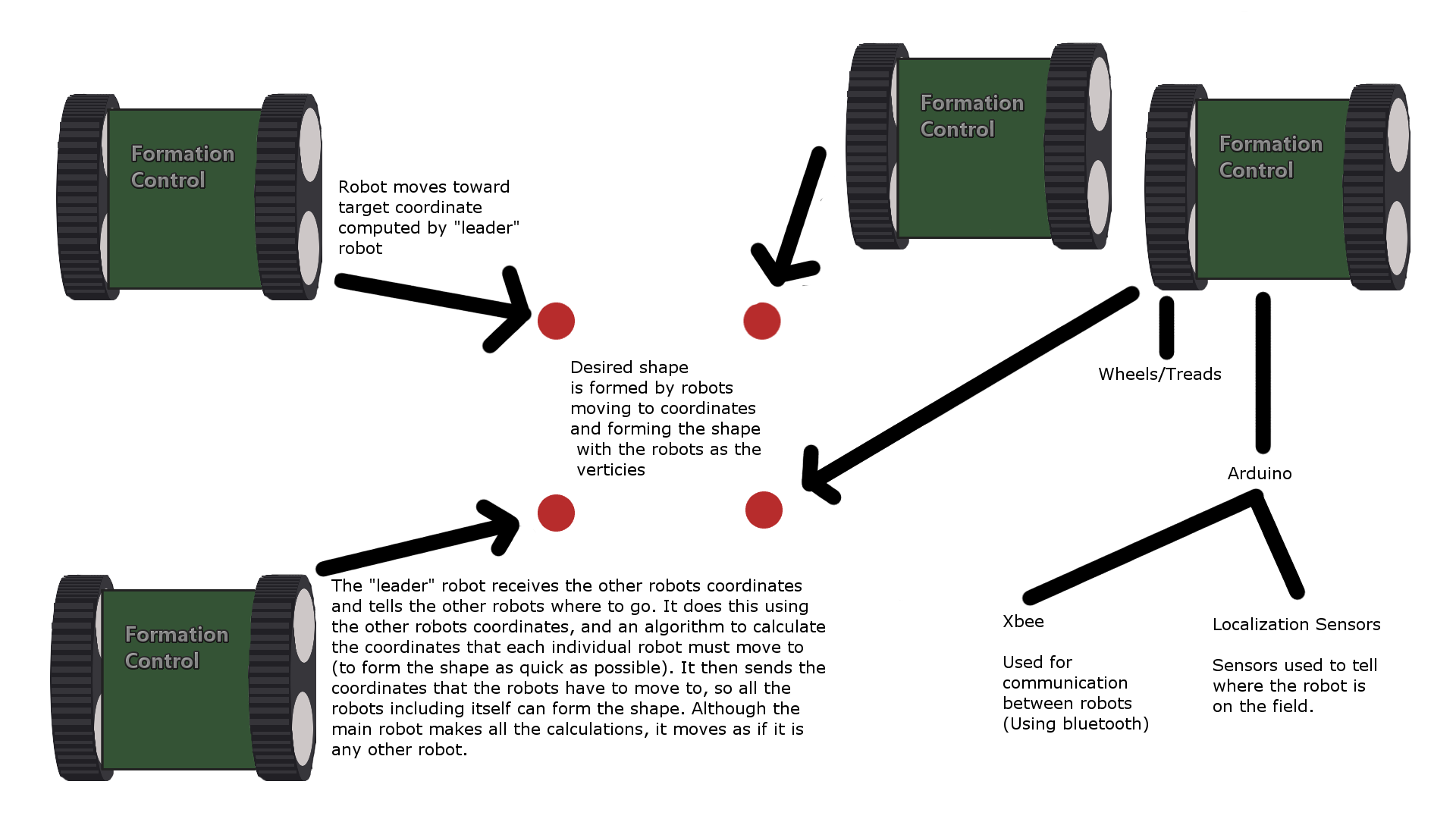# Software ArchitectureClick to enlarge Coordinate Evaluation: Each robot’s polar coordinate(compass heading and distance to beacon) is converted to Cartesian. If the robot coordinates are concave, the convex hull of the coordinates is determined using Jarvis March to get a convex shape. Polygon Formation: The centroid of the convex hull is calculated. The coordinates of the polygon are calculated using the centroid as its center and the input side length. Point Assignment: Each robot’s distance to the points of the polygon are calculated and ranked sequentially The first robot is assigned the point on the polygon closest to it, the next robot chooses the next closest point, and so on… Polygon Optimization: To maximize the shortest distances and minimize the longest ones, the coordinates of the polygon are moved and distances to the robots are recalculated until the average distance approaches the target.